# Estimators (DL4 to DL5, and DL6)#

The gammapy.estimators submodule contains algorithms and classes for high level flux and significance estimation. This includes estimation flux points, flux maps, flux points, flux profiles and flux light curves. All estimators feature a common API and allow estimating fluxes in bands of reconstructed energy.

## General method#

The core of any estimator algorithm is hypothesis testing: a reference model or counts excess is tested against a null hypothesis. From the best fit reference model a flux is derived and a corresponding $$\Delta TS$$ value from the difference in fit statistics to the null hypothesis. Assuming one degree of freedom, $$\sqrt{\Delta TS}$$ represents an approximation (Wilk’s theorem) of the “classical significance”. In case of a negative best fit flux, e.g. when the background is overestimated, the significance is defined as $$-\sqrt{\Delta TS}$$ by convention.

In general the flux can be estimated using two methods:

1. Based on model fitting: given a (global) best fit model with multiple model components, the flux of the component of interest is re-fitted in the chosen energy, time or spatial region. The new flux is given as a norm with respect to the global reference model. Optionally other component parameters in the global model can be re-optimised. This method is also named forward folding.

2. Based on excess: in the case of having one energy bin, neglecting the PSF and not re-optimising other parameters, one can estimate the significance based on the analytical solution by [LiMa1983]. In this case the “best fit” flux and significance are given by the excess over the null hypothesis. This method is also named backward folding. This method is currently only exposed in the ExcessMapEstimator

## Flux quantities#

In case the data is fitted to a single data bin only, e.g. one energy bin Uniformly for both methods most estimators compute the same basic quantities:

Quantity

Definition

norm

Best fit norm with respect to the reference spectral model

norm_err

Symmetric error on the norm derived from the Hessian matrix. Given as absolute difference to the best fit norm.

stat

Fit statistics value of the best fit hypothesis

stat_null

Fit statistics value of the null hypothesis

ts

Difference in fit statistics (stat - stat_null )

sqrt_ts

Square root of ts time sign(norm), in case of one degree of freedom, corresponds to significance (Wilk’s theorem)

npred

Predicted counts of the best fit hypothesis. Equivalent to correlated counts for backward folding

npred_excess

Predicted excess counts of the best fit hypothesis. Equivalent to correlated excess for backward folding

npred_background

Predicted background counts of the best fit hypothesis. Equivalent to correlated excess for backward folding

In addition, the following optional quantities can be computed:

Quantity

Definition

norm_errp

Positive error of the norm, given as absolute difference to the best fit norm

norm_errn

Negative error of the norm, given as absolute difference to the best fit norm

norm_ul

Upper limit of the norm

norm_scan

Norm scan

stat_scan

Fit statistics scan

To compute the error, asymmetric errors as well as upper limits one can specify the arguments n_sigma and n_sigma_ul. The n_sigma arguments are translated into a TS difference assuming ts = n_sigma ** 2.

In addition to the norm values a reference spectral model and energy ranges are given. Using this reference spectral model the norm values can be converted to the following different SED types:

Quantity

Definition

e_ref

Reference energy

e_min

Minimum energy

e_max

Maximum energy

dnde

Differential flux at e_ref

flux

Integrated flux between e_min and e_max

eflux

Integrated energy flux between e_min and e_max

e2dnde

Differential energy flux at e_ref

The same can be applied for the error and upper limit information. More information can be found on the likelihood SED type page.

The FluxPoints and FluxMaps objects can optionally define meta data with the following valid keywords:

Name

Definition

n_sigma

Number of sigma used for error estimation

n_sigma_ul

Number of sigma used for upper limit estimation

ts_threshold_ul

TS threshold to define the use of an upper limit

A note on negative flux and upper limit values:

Note

Gammapy allows for negative flux values and upper limits by default. While those values are physically not valid solutions, they are still valid statistically. Negative flux values either hint at overestimated background levels or underestimated systematic errors in general. Or in case of many measurements, such as pixels in a flux map, they are even statistically expected. For flux points and light curves the amplitude limits (if defined) are taken into account. In future versions of Gammapy it will be possible to account for systematic errors in the likelihood as well. For now the correct interpretation of the results is left to the user.

## Flux maps#

This how to compute flux maps with the ExcessMapEstimator:

import numpy as np
from gammapy.datasets import MapDataset
from gammapy.estimators import ExcessMapEstimator
from astropy import units as u

dataset = MapDataset.read("$GAMMAPY_DATA/cta-1dc-gc/cta-1dc-gc.fits.gz") estimator = ExcessMapEstimator( correlation_radius="0.1 deg", energy_edges=[0.1, 1, 10] * u.TeV ) maps = estimator.run(dataset) print(maps["flux"])  WcsNDMap <BLANKLINE> geom : WcsGeom axes : ['lon', 'lat', 'energy'] shape : (320, 240, 2) ndim : 3 unit : 1 / (cm2 s) dtype : float64 <BLANKLINE>  ## Flux points# This is how to compute flux points: from astropy import units as u from gammapy.datasets import SpectrumDatasetOnOff, Datasets from gammapy.estimators import FluxPointsEstimator from gammapy.modeling.models import PowerLawSpectralModel, SkyModel path = "$GAMMAPY_DATA/joint-crab/spectra/hess/"

datasets = Datasets([dataset_1, dataset_2])

pwl = PowerLawSpectralModel(index=2, amplitude='1e-12  cm-2 s-1 TeV-1')

datasets.models = SkyModel(spectral_model=pwl, name="crab")

estimator = FluxPointsEstimator(
source="crab", energy_edges=[0.1, 0.3, 1, 3, 10, 30, 100] * u.TeV
)

# this will run a joint fit of the datasets
fp = estimator.run(datasets)
table = fp.to_table(sed_type="dnde", formatted=True)
# print(table[["e_ref", "dnde", "dnde_err"]])

# or stack the datasets
# fp = estimator.run(datasets.stack_reduce())
table = fp.to_table(sed_type="dnde", formatted=True)
# print(table[["e_ref", "dnde", "dnde_err"]])


## Using gammapy.estimators#

### Examples using gammapy.estimators.FluxPointsEstimator#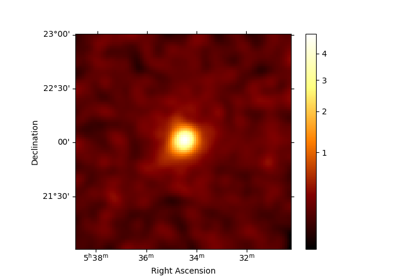Low level API

Low level API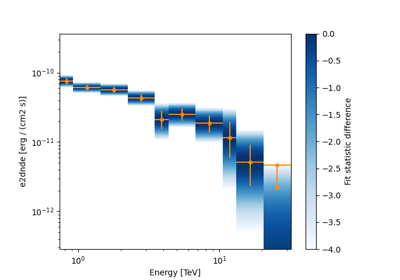Spectral analysis

Spectral analysis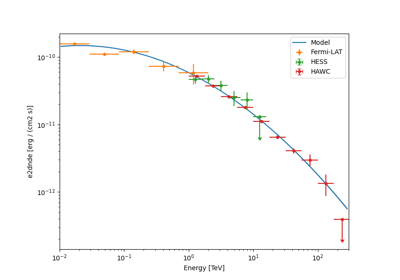Multi instrument joint 3D and 1D analysis

Multi instrument joint 3D and 1D analysis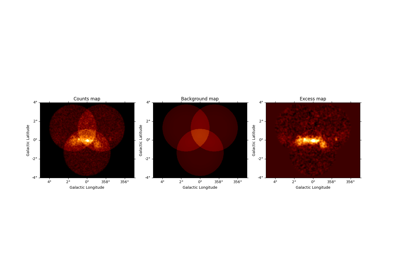Basic image exploration and fitting

Basic image exploration and fitting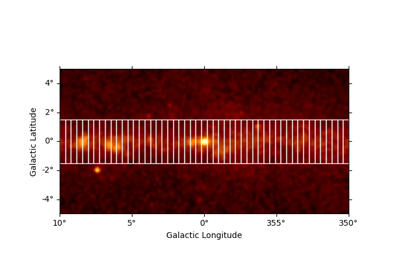Flux Profile Estimation

Flux Profile Estimation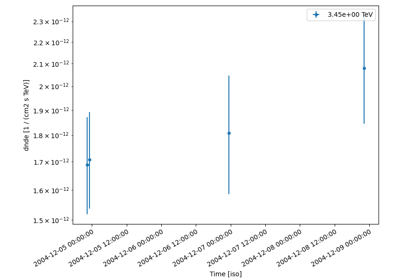Light curves

Light curves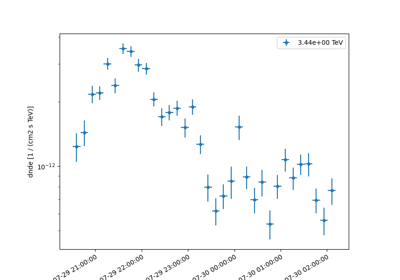Light curves for flares

Light curves for flares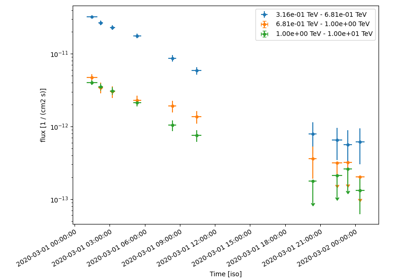Simulating and fitting a time varying source

Simulating and fitting a time varying source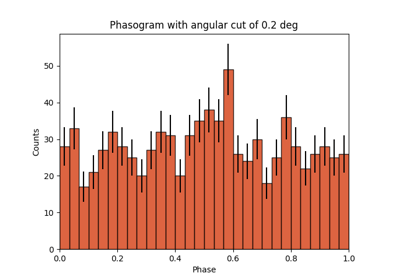Pulsar analysis

Pulsar analysis

### Examples using gammapy.estimators.LightCurveEstimator#Light curves

Light curvesLight curves for flares

Light curves for flaresSimulating and fitting a time varying source

Simulating and fitting a time varying source